Courses

# RD Sharma Solutions -Ex-7.10, Decimals, Class 6, Maths Class 6 Notes | EduRev

## RD Sharma Solutions for Class 6 Mathematics

Created by: Abhishek Kapoor

## Class 6 : RD Sharma Solutions -Ex-7.10, Decimals, Class 6, Maths Class 6 Notes | EduRev

The document RD Sharma Solutions -Ex-7.10, Decimals, Class 6, Maths Class 6 Notes | EduRev is a part of the Class 6 Course RD Sharma Solutions for Class 6 Mathematics.
All you need of Class 6 at this link: Class 6

1.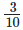is equals to

c) 0.3

2.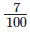is equals to

d) 0.07

3.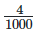is equals to

0.004

4. The value of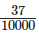is:

0.0037

5. The place value of 5 in 0.04532 is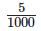6. The value of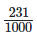0.231

7. The value of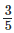100

3.005

8. The value of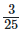0.12

9. The value of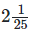2.04

10.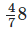is equals to

c)4.875

11. 2+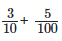is equals to

is equals to

c)2.35

12.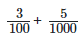is equals to

c)0.0305

13. 1 cm is equals to

0.01 m

14. 1 m is equals to

c)0.001 km

15. 2 kg 5 gm is equal to

2.005 kg

16. 15 litres and 15 ml is equals to

d ) 15.015 litres

17. Which of the following are like decimals

c)5.5,6.6,7.7,8.8

18. The value of the 0.5+0.005+5.05 is

b) 5.555

19. 0.35-0.035 is equal to

c)0.0315

20. 2.5+3.05-4.005 is equals to

1.545

21. Which is greater among 2.3 , 2.03,2.33, 2.05?

c)2.33

Offer running on EduRev: Apply code STAYHOME200 to get INR 200 off on our premium plan EduRev Infinity!

103 docs

,

,

,

,

,

,

,

,

,

,

,

,

,

,

,

,

,

,

,

,

,

,

,

,

,

,

,

,

,

,

;